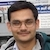# NAEST 2016 Screening Test: Resistance of a WireBy

The video shows two wires, white and red, of almost equal lengths. The resistivity and cross-sectional area of the white wire are ρ1 and A1. For the red wire, the resistivity and cross-sectional area are ρ2 and A2. These wires are connected to a battery. The current through the white and the red wires are I1 and I2.

Question: The resistivity of white wire is $\rho_1$ and its cross-sectional area is $A_1$. The resistivity of the red wire is $\rho_2$ and its cross-sectional area is $A_2$. The current through these wires are $I_1$ and $I_2$. Choose the correct option(s):

1. $I_1 = I_2$
2. $I_1/A_1 = I_2/A_2$
3. $I_1/\rho_1 = I_2/\rho_2$
4. $I_1/ (\rho_1 A_1) = I_2/ (\rho_2 A_2)$

Solution: Observe that wires are connected in series. Thus, equal current flows through the two wires i.e., $I_1=I_2$.

The resistances of these wires of equal lengths $l$ are given by \begin{align} R_1&=\rho_1 l/A_1, \\ R_2&=\rho_2 l/A_2. \end{align} The equivalent resistance of wires connected in series is \begin{align} R_{eq}&=R_1+R_2\\ &=\left(\frac{\rho_1}{A_1}+\frac{\rho_2}{A_2}\right)l \end{align}

Generally, the resistivity of connecting wire material is low (conductivity is high). The resistances $R_1$ and $R_2$ for given wires will be very low. A large current flow through the wires if they are directly connected across a battery (as in video). The high current leads to higher joule heating ($i^2 R t$) and a sharp rise in the wire's temperature. This also drains the battery very fast. Connecting wire should not be directly connected across a battery.